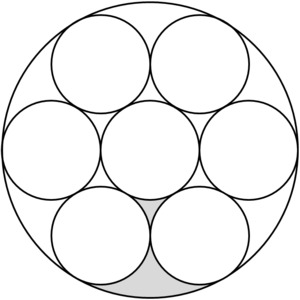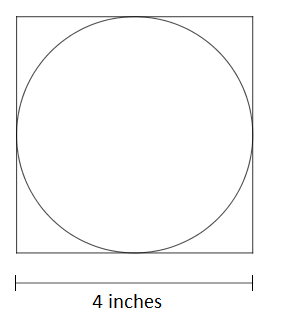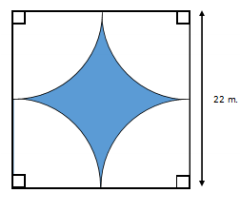# Geometry

## Objective

Solve problems involving area and circumference of two-dimensional figures (Part 2).

## Common Core Standards

### Core Standards

?

• 7.G.B.4 — Know the formulas for the area and circumference of a circle and use them to solve problems; give an informal derivation of the relationship between the circumference and area of a circle.

• 7.G.B.6 — Solve real-world and mathematical problems involving area, volume and surface area of two- and three-dimensional objects composed of triangles, quadrilaterals, polygons, cubes, and right prisms.

?

• 6.G.A.1

• 7.G.A.1

## Criteria for Success

?

1. Know and use the formulas for area and circumference of a circle.
2. Use a scale to find the actual area and perimeter/circumference from scale drawings.
3. Find the area and perimeter/circumference of composite shapes and shaded regions, including shapes composed of circles, semi-circles, quadrilaterals, and triangles.

## Tips for Teachers

?

• Lessons 10 and 11 engage students in finding area and circumference/perimeter measurements of different geometric shapes including circles, quadrilaterals, and triangles. The Problem Set Guidance includes a variety of problems that range in difficulty and time required.
• Students engage in MP.1 and MP.7 as they make sense of complex geometric figures, looking for significance of shapes and measurements within the structure of the diagram, and looking for entry points to solve the problem. Support students by asking questions about what they observe in the diagrams and what initial strategy ideas they have.

#### Fishtank Plus

• Problem Set
• Student Handout Editor
• Vocabulary Package

## Anchor Problems

?

### Problem 1

The figure below is composed of eight circles, seven small circles and one large circle containing them all. Neighboring circles only share one point, and two regions between the smaller circles have been shaded. Each small circle has a radius of 5 cm.Calculate:

1. The area of the large circle.
2. The area of the shaded part of the figure.

#### References

Illustrative Mathematics Eight Circles

Eight Circles, accessed on June 2, 2017, 11:46 a.m., is licensed by Illustrative Mathematics under either the CC BY 4.0 or CC BY-NC-SA 4.0. For further information, contact Illustrative Mathematics.

### Problem 2

A circular mirror is placed in a square wooden frame, as shown in the scale drawing below. The scale of the drawing is 1 inch to 6 inches.1. What is the actual perimeter of the wooden frame?
2. What is the actual area of the circular mirror?

## Problem Set

?The following resources include problems and activities aligned to the objective of the lesson that can be used to create your own problem set.

?

The unshaded regions are quarter circles. Approximate the area of the shaded region. Use 3.14 for ${\pi}$.#### References

EngageNY Mathematics Grade 7 Mathematics > Module 3 > Topic C > Lesson 20Exit Ticket

Grade 7 Mathematics > Module 3 > Topic C > Lesson 20 of the New York State Common Core Mathematics Curriculum from EngageNY and Great Minds. © 2015 Great Minds. Licensed by EngageNY of the New York State Education Department under the CC BY-NC-SA 3.0 US license. Accessed Dec. 2, 2016, 5:15 p.m..

Modified by The Match Foundation, Inc.

?